MORE IN Structural Analysis - 1
GTU Civil Engineering (Semester 3)
Structural Analysis - 1
May 2014
Total marks: --
Total time: --
INSTRUCTIONS
(1) Assume appropriate data and state your reasons
(2) Marks are given to the right of every question
(3) Draw neat diagrams wherever necessary

1 (a) (i) Write the assumptions made in the theory of pure torsion.
3 M
1 (a) (ii) Write the equations for Euler's crippling load for different end conditions of a long column.
4 M
1 (b) (i) "Indeterminate structures are better than determinate structures" Comment on the statement.
3 M
1 (b) (ii) A suspension cable having supports at the same level, has a span of 30m and a maximum dip of 3m. The cable is loaded with a uniformly distributed load of 10 kN/m through out its length. Find the maximum tension in the cable.
4 M

2 (a) (i) Differentiate between the real beam and conjugate beam.
3 M
2 (a) (ii) Differentiate between the terms : beam, truss, frame and arch.
4 M
2 (b) A hollow shaft has to transmit 300kW power at 80 RPM. If the shear stress is not to exceed 60 N/mm 2 and internal diameter is 60% of the external diameter, find the external and internal diameters when maximum torque is 1.4 times the average torque. G= 8 × 104 N/mm2.
7 M
2 (c) A solid shaft transmits 90 kW at 180 RPM. The maximum shear stress is limited to 60 N/mm2 . Determine the length of the shaft if the twist in the shaft must not exceed 1° over its entire length. Take G= 8 × 104 N/mm2 .
7 M

3 (a) Derive the equation for strain energy stored in an element due to bending. Also find the deflection at the free end of a cantilever beam subjected to a point load at the free end with constant EI by this method.
7 M
3 (b) Find the slope and deflection at the free end B of a cantilever beam AB as shown in fig.(i) by moment area method. Take I= 2 × 108 mm4 , E= 2 × 105 N/mm2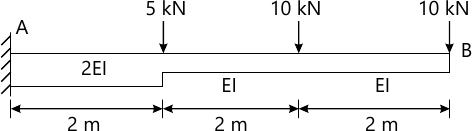7 M
3 (c) A load of 500 N falls freely through a height of 150 mm on to a collar attached to the end of a vertical rod of 50 mm diameter and 2m long. The upper end of the rod is fixed Calculate the maximum instantaneous extension of the bar. Also calculate maximum stress in the bar. E= 2 × 105 N/mm2
7 M
3 (d) Find the slope and deflection at the centre C of a simply supported beam AB as shown in fig.(ii) by moment area method. Take I= 2 × 108 mm4 , E= 2 × 105 N/mm2.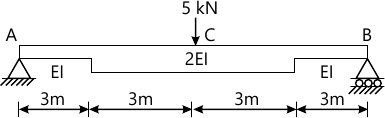7 M

4 (a) A symmetrical three hinged parabolic arch of span 40 m and rise 8m carries uniformly distributed load of 30 kN/m over the left half of the span. The hinges are provided at the support and centre of the arch. Calculate the bending moment, radial shear and normal thrust at a distance of 10m from the left support. Refer fig.(iii)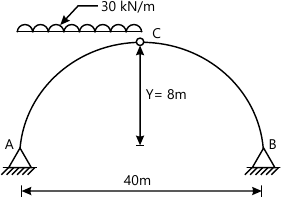7 M
4 (b) A simply supported beam of 3m span carries two point loads of 120 kN and 80 kN at a distance of 0.6m and 2 m from the left support. If for the beam I = 16 × 108 mm 4 and E = 2.1 × 105 N/mm2 , calculate the deflection under loads using Macaulay's method. Refer fig.(iv)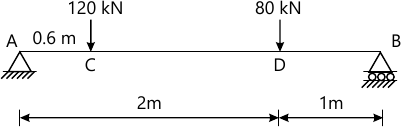7 M
4 (c) A cylindrical vessel closed with plane ends is made of a 4mm thick steel plate. Its external diameter is 250 mm and length is 750 mm. It is subjected to an internal fluid pressure of 3 N/mm2 , calculate the longitudinal and hoop stresses in the shell plate. Also calculate change in diameter, length and volume of the cylinder. Take E = 2.1 × 105 N/mm2 , Poisson's ratio = 0.3.
7 M
4 (d) A rectangular pier of size 300mm × 300mm is subjected to a compressive load of 900 kN at one of the corner. Find the stress intensities at all four corners of the pier and draw stress distribution diagram.. If the load is acting at the center of the pier, also draw the stress distribution diagram.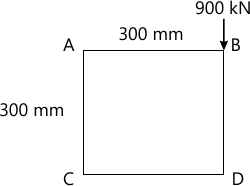7 M

5 (a) A hollow cast iron column has out side diameter 200mm and thickness of 20mm. It is 4.5m long and fixed at both ends .Calculate the safe load and ratio of Euler's and Rankine's critical load. For cast iron F c = 550N/mm2 , α =1/1600 and E= 0.8 × 105 N/mm2.
7 M
5 (b) A concrete dam of trapezoidal section has a top width 2m ,bottom width of 6m and height 12m. It retains water up to 10 m on vertical side. Determine the maximum and minimum stress intensities at the base of the section. The density of the material used is 24 kN/m3 and density of water as 10 kN/m3 .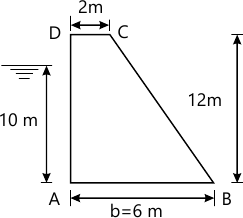7 M
5 (c) uniformly distributed load of 12kN/m and 3m length crosses a simply supported girder of span 10m from left to right. Draw influence line for shear force and bending moment at 4m from left hand and find maximum shear force and bending moment at this section.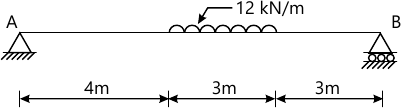7 M
5 (d) Prove that for a three hinged parabolic arch of span L and rise h, subjected to a uniformly distributed load, the bending moment at any section is zero.
7 M

More question papers from Structural Analysis - 1